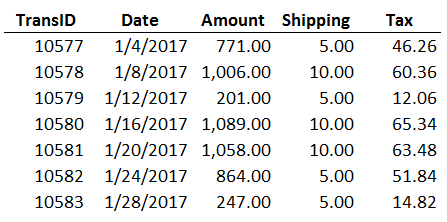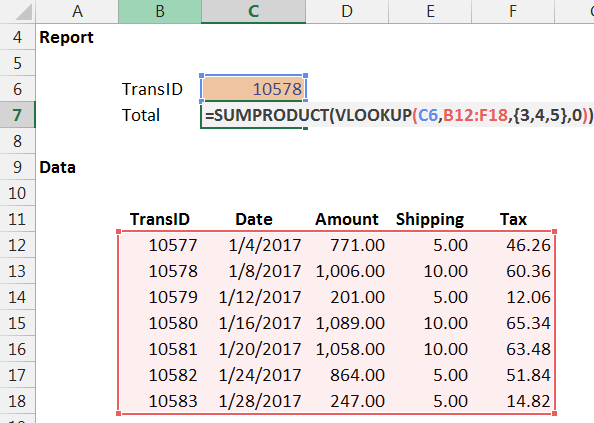## Return the Sum of Two or More Columns with VLOOKUP

If you have ever wished that the VLOOKUP function could return the sum of two or more related columns, this trick will get you there.

## Objective

Before we get into the details, let’s be clear about our objective. We have some transactions that were exported from our accounting system as shown below.We would like to write a formula that will look up any given TransID and return the sum of three columns, Amount, Shipping, and Tax. This is illustrated in the screenshot below, where we enter a TransID such as 10578 and Excel computes the Total (1,076.36 = 1,006 + 10 + 60.36).Since this is essentially a lookup task, our first instinct is to use VLOOKUP. However, we know that VLOOKUP can only return one related value, not the sum of multiple related values.

One common workaround is to add a helper column to the data that sums the three columns and then use a VLOOKUP to return the value from the new helper column. However, whenever possible in practice, we prefer to work with the data as it comes so that the workbook is easy to update in future periods.

Another common workaround is to write a formula that adds up three VLOOKUP functions, such as the following.

`=VLOOKUP(...) + VLOOKUP(...) + VLOOKUP(...)`

However, such a formula can be difficult to update and maintain over time since any change to the arguments need to be made three times instead of once. The good news is that we can wrap a SUMPRODUCT function around a single VLOOKUP function. Let’s get started.

## SUMPRODUCT

When the SUMPRODUCT has a single argument, it behaves much like the SUM function because it simply returns the sum of the values. When the SUMPRODUCT has multiple arguments, it returns the sum of the product of its arguments. To accomplish our objective, we will use the SUMPRODUCT to return the sum of the values in a single argument.

Basically, we will wrap a SUMPRODUCT function around a VLOOKUP function that returns an array of values, specifically, the related values from multiple columns. The SUMPRODUCT will sum the values in the array returned by the VLOOKUP function. How do we convince the VLOOKUP function to return an array of values? That brings us to our next discussion.

Technical Note: we need to use SUMPRODUCT instead of SUM because the SUMPRODUCT function is designed to work with arrays and will thus return the sum of the array returned by the VLOOKUP function. The SUM function would return the sum of the first array element only. However, you could use the SUM function and then array-enter it with Control+Shift+Enter if preferred.

## Array of Values

We can ask the VLOOKUP function to return an array of values by enclosing the third argument in curly braces {}. This causes the VLOOKUP function to actually return an array that contains multiple elements. For example, if we wanted the VLOOKUP function to return the values from the 3rd, 4th, and 5th columns to an array with three elements, we could use the following formula.

`=VLOOKUP(C6,B12:F18,{3,4,5},0)`

Where:

• C6 is the lookup value
• B12:F18 is the lookup range
• {3,4,5} are the column numbers that have the values we want returned, enclosed in curly braces to create the array of values
• 0 tells VLOOKUP to use exact match logic

This VLOOKUP function actually returns an array of values, and the array elements are 1006, 10, and 60.36. If you enter it into a single cell and press Enter, Excel will display only the first element. But, we don’t want to display the first element, we want to compute the sum of all array elements. So, let’s put it all together and bring it home.

Technical note: you can confirm the VLOOKUP returns an array of values by array-entering the formula into three cells across at once (Ctrl+Shift+Enter). Excel will display the three array elements in the three cells.

## SUMPRODUCT and VLOOKUP

Now that we understand that using the curly braces causes VLOOKUP to return an array of values, let’s add up the values in the returned array with the SUMPRODUCT function.

Here is a screenshot of our workbook.The formula in C7 follows.

`=SUMPRODUCT(VLOOKUP(C6,B12:F18,{3,4,5},0))`

The SUMPRODUCT function returns the sum of the array elements returned by the VLOOKUP function. This is essentially the same as SUMPRODUCT({1006, 10, 60.36}), which returns 1076.36.

When we press Enter, we confirm that it works as expected…yes!Note: there is no need to array-enter this formula by using Control-Shift-Enter.

And that my friend is one way to return the sum of two or more columns with VLOOKUP. If you have another approach, please share by posting a comment below…thanks!

Posted in ,### Jeff Lenning

I love sharing the things I've learned about Excel, and I built Excel University to help me do that. My motto is: Learn Excel. Work Faster.

### Stay Connected

1.joe szczepaniak on October 29, 2015 at 1:10 pm

That’s really clever! How did you run across the option of putting an array of columns into the VLOOKUP function?

•jefflenning on October 30, 2015 at 6:06 am

Joe,
That technique came from a participant in a discussion during one of my Excel sessions…pretty cool!
There is so much to learn about Excel…I wish I had discovered that trick years ago 🙂
Thanks
Jeff

2.Jon D Schaefer on May 19, 2016 at 8:21 am

Excellent – thank you. Is there any way to replace the {3,4,5} part with a name? Like can I name a group of columns and reference them that way? Not finding anything about naming an array.

•Kurt LeBlanc on July 27, 2016 at 8:55 am

Hey Jon,

You can create a named formula, as in ={3,4,5}, and use that instead:)

Let me know if you need anymore help!
Kurt LeBlanc

3.Nick on May 31, 2016 at 4:05 pm

Jeff, this is a really cool solution.
More to the point – you explain it clearly and succinctly in a way that is not only easy to follow, but helps to ‘stick’ it in the brain.

Nick

•jefflenning on May 31, 2016 at 4:37 pm

Thanks 🙂

4.jain_tech on June 23, 2016 at 9:25 pm

Will this similarly work with hlookup?

•Kurt LeBlanc on June 30, 2016 at 1:57 pm

Correct! It works because SUMIFS() works horizontally as well as vertically.

5.Srikanth on August 11, 2016 at 9:21 pm

Thanks for the useful information.. I would like to add multiple values which are in the same column (unlike the example here where the addition is along the same row)…How do I do that. Can you please suggest.?

•Kurt LeBlanc on August 16, 2016 at 12:13 pm

Hey Srinkanth!

Absolutely, with HLOOKUP:) It is exactly the same logic fortunately, just with nth row as the third argument instead of column. If you transpose the table in the sample file and adjust the lookup formula to HLOOKUP(), you can see hat even the array works as expected:)

Let me know if you need any help with the setup,
Kurt LeBlanc

•shaibajit on September 5, 2016 at 3:31 am

Hi Kurt,
My table area is A1:K30. My Lookup value matches with H1. And I want to add from H10 to H28. Instead of writing all the row numbers from 10 to 28 withing HLookup [ like – hlookup{10,11,12,13,…….,26,27,28} ] is there any other options?

•Kurt LeBlanc on September 6, 2016 at 8:19 am

I understand now:)

Unfortunately, you have to enter each of the individual numbers in the array…or you can create a named formula that refers to the array, and use that in your lookup.

Let me know if that is clear
Kurt LeBlanc

6.Adrian Ramirez on October 14, 2016 at 12:24 pm

This post is to subscribe to follow-up emails (meant to do that earlier, but forgot)

•jefflenning on October 14, 2016 at 12:32 pm

Great…thanks!

7.Sam on November 7, 2016 at 10:28 pm

Thanks this is a great post. However, what’s missing is the solution that sums up multiple values in one column. All the values I want to sum up are not in multiple columns but in the same column. How do I make this work? Thanks in advance

8.Janeske on June 19, 2017 at 2:23 am

Good day,
I really benefit from this – Thank you!!
Just a question I have a vlookup table A:Q3229 and needs to sum the months from Jan to June (depending on the month). Please see my formula below:
{=IFERROR(SUM(VLOOKUP(E18,budget!A:Q,{5,6,7,8,9,10},0)),””)} this formula is working perfectly – however is there a way if you change the month in the input sheet that it can add a column (Jan to July) to add instead of adding column 11 to my formula?
I would like to sent the sheet out to individuals to fill in and they won’t be able to change the formula.

•Jeff Lenning on June 19, 2017 at 4:18 am

I’d probably suggest using SUMIFS instead of VLOOKUP, which supports the use of comparison operators (such as > and <) in the criteria arguments. I've written a bunch of posts on SUMIFS if you haven't used it before: http://www.excel-university.com/tag/sumifs/

Hope it helps,
Jeff

9.Luis Alberto Siles Tapia on June 30, 2017 at 8:00 pm

Hello,
If I where to have multiple “lookup values” in a table and I´d like the sum of all ID “a”
ID
a 3 4 1
b 4 5 2
c 6 1 5
a 7 2 0
d 1 1 9
a 0 1 7

ID a
Total = 3+4+1+7+2+0+0+1+7
How could I achive this? using the formula it only gives me: 3+4+1

10.Mantu Singh on May 25, 2018 at 1:16 pm

Very useful post for Excel users. Thanks a lot for this post.

11.Tuan Nguyen on August 21, 2018 at 9:39 am

Hello Jeff,

Thank you for the incredibly useful article!

I have a unique situation and I was wondering if you could help me out. I have monthly income statement data (from Jan-Dec) in one tab and an output page on another tab. On the output page, I have two cells – beginning month and ending month; which reflects the performance period I want to look at. I.e. if I wanted to see the performance for January thru June, I would enter 1 and 6 in the respective cells.

My question is: is there any way I can sum the revenues (as an example) for the different columns by using a combination of vlookup and sumproduct? I can’t use the technique you described because the column numbers here are hard-coded whereas I am trying to make my output page more dynamic so I don’t have to go in and change the formula every time.

12.FM on January 28, 2020 at 11:06 am

Many thanks, but could I simply things;
=SUMPRODUCT((\$G\$5:\$I\$11)*(\$L\$4=\$E\$5:\$E\$11))) = 1076.36

simply using sumproduct without a lookup function will work as above where cell L4 = 10578
There are many ways, just thought I would add another!
Kind regards

•Jeff Lenning on January 28, 2020 at 11:23 am

Nice … thanks!

13.theo tran on March 3, 2020 at 11:54 am

very good !.

!
!